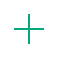# 如何解决python中递归层数过多的异常？

Python中递归函数的调用层数过深，引发异常，报错信息如下所示：

`RuntimeError: maximum recursion depth exceeded`Mar 1, 2019
1 赞

Python中递归函数的层数是有限的；这算是对系统资源的一种保护机制。

```import sys
sys.setrecursionlimit(10000) # 10000 is just an example```

```def factorial(n):
if n == 1:
return 1
return n * factorial(n-1)```

```def factorial(n):
return factorial_iteration(n, 1)

def factorial_iteration(n, r):
if n == 1:
return r
return factorial_iteration(n - 1, n * r)```